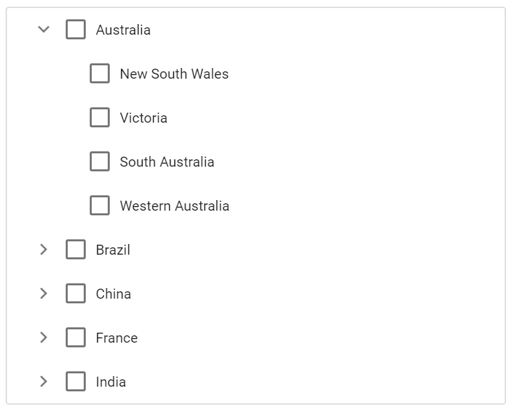Search results

CheckBox in ASP.NET MVC TreeView control

18 Jan 2022 / 2 minutes to read

The TreeView control allows you to check more than one node in TreeView without affecting the UI’s appearance by enabling the showCheckBox property. When this property is enabled, checkbox appears before each TreeView node text.

• If one of the child nodes is not in a checked state, then the parent node will be in an intermediate state.
• If all the child nodes are in checked state, then the parent node’s state will also be checked.
• If a parent node is checked, then all the child nodes’ state will also be checked.

By default, the checkbox state of parent and child nodes are dependent on each other. If you need independent checked state, you can achieve it using the autoCheck property.

Using the checkedNodes property, you can set the nodes that need to be checked or get the ID of nodes that are currently checked in the TreeView control.

If you need to prevent the node check action for a particular node, the nodeChecking event can be used which is triggered before the TreeView node is checked/unchecked. The nodeChecked event will be triggered when the TreeView node is checked/unchecked successfully.

In the following example, the showCheckBox property is enabled.

razor
checkbox.cs
@Html.EJS().TreeView("treedata").ShowCheckBox(true).Fields(field=>
field.Id("id").ParentID("pid").Text("name").HasChildren("hasChild").Expanded("expanded")
.DataSource(ViewBag.dataSource)).Render()
public IActionResult CheckBox()
{
List<object> treedata = new List<object>();
{
id = 1,
name = "Australia",
hasChild = true,
expanded = true
});
{
id = 2,
pid = 1,
name = "New South Wales",

});
{
id = 3,
pid = 1,
name = "Victoria"
});

{
id = 4,
pid = 1,
name = "South Australia"
});
{
id = 6,
pid = 1,
name = "Western Australia",

});
{
id = 7,
name = "Brazil",
hasChild = true
});
{
id = 8,
pid = 7,
name = "Paraná"
});
{
id = 9,
pid = 7,
name = "Ceará"
});
{
id = 10,
pid = 7,
name = "Acre"
});
{
id = 11,
name = "China",
hasChild = true
});
{
id = 12,
pid = 11,
name = "Guangzhou"
});
{
id = 13,
pid = 11,
name = "Shanghai"
});
{
id = 14,
pid = 11,
name = "Beijing"
});
{
id = 15,
pid = 11,
name = "Shantou"

});
{
id = 16,
name = "France",
hasChild = true

});
{
id = 17,
pid = 16,
name = "Pays de la Loire"

});
{
id = 18,
pid = 16,
name = "Aquitaine"

});
{
id = 19,
pid = 16,
name = "Brittany"

});
{
id = 20,
pid = 16,
name = "Lorraine"
});
{
id = 21,
name = "India",
hasChild = true

});
{
id = 22,
pid = 21,
name = "Assam"

});
{
id = 23,
pid = 21,
name = "Bihar"
});
{
id = 24,
pid = 21,

});
ViewBag.dataSource = treedata;
return View();
}

Output be like the below.Checked nodes

You can get or set the checked nodes in TreeView at initial rendering and dynamically by using the checkedNodes property. It returns the checked nodes’ ID as an array.

In the following example, the New South Wales and Western Australia nodes are checked at initial rendering. If any more nodes are checked, the checked nodes’ IDs will be displayed in alert.

razor
checkbox.cs
@Html.EJS().TreeView("treedata").ShowCheckBox(true).NodeChecked("nodeChecked").Fields(field=>
field.Id("id").ParentID("pid").Text("name").HasChildren("hasChild").Expanded("expanded")
.DataSource(ViewBag.dataSource)).CheckedNodes(ViewBag.checkedNodes).Render()
<script>
function nodeChecked(args) {
alert("The checked node's id: " + this.checkedNodes); // To alert the checked node's id.
}
</script>
public IActionResult CheckBox()
{
List<object> treedata = new List<object>();
{
id = 1,
name = "Australia",
hasChild = true,
expanded = true
});
{
id = 2,
pid = 1,
name = "New South Wales",

});
{
id = 3,
pid = 1,
name = "Victoria"
});

{
id = 4,
pid = 1,
name = "South Australia"
});
{
id = 6,
pid = 1,
name = "Western Australia",

});
{
id = 7,
name = "Brazil",
hasChild = true
});
{
id = 8,
pid = 7,
name = "Paraná"
});
{
id = 9,
pid = 7,
name = "Ceará"
});
{
id = 10,
pid = 7,
name = "Acre"
});
{
id = 11,
name = "China",
hasChild = true
});
{
id = 12,
pid = 11,
name = "Guangzhou"
});
{
id = 13,
pid = 11,
name = "Shanghai"
});
{
id = 14,
pid = 11,
name = "Beijing"
});
{
id = 15,
pid = 11,
name = "Shantou"

});
{
id = 16,
name = "France",
hasChild = true

});
{
id = 17,
pid = 16,
name = "Pays de la Loire"

});
{
id = 18,
pid = 16,
name = "Aquitaine"

});
{
id = 19,
pid = 16,
name = "Brittany"

});
{
id = 20,
pid = 16,
name = "Lorraine"
});
{
id = 21,
name = "India",
hasChild = true

});
{
id = 22,
pid = 21,
name = "Assam"

});
{
id = 23,
pid = 21,
name = "Bihar"
});
{
id = 24,
pid = 21,